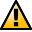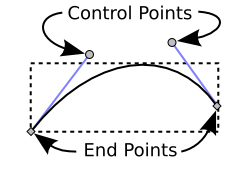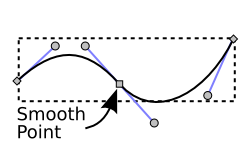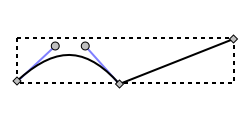WarningThis section not yet updated for Inkscape v0.92.

## Bezier Curves

Most paths are described internally in Inkscape (and in many other drawing programs) as a series of Bezier curves. It is very useful to understand the basic properties of Bezier curves for drawing and manipulating paths. Bezier curves are defined by four points, two of which are the end points or nodes of the curve. The other two are control points or handles, each paired with one of the end points. The control points have the useful property that a line starting at one end of the curve and ending at the corresponding control point is tangent to the curve at the end point. This enables the smooth joining of multiple Bezier curves to form a path.A Bezier curve showing the end points (nodes) and control points (handles).

Two or more Bezier curves can be joined to form a more complex path. The node where they are joined may be smooth, indicated by a square (normal) or circle (auto-smooth node, see Auto-smooth Nodes).Two Bezier curves joined by a smooth node.

Or the node may be a corner node, also referred to as a cusp node, indicated by a diamond, where an abrupt change in direction is allowed.Two Bezier curves joined by a corner node.

The segment between two nodes may be a curve or a line. Note that there are no control points for line segments.Two segments, one a curve and the other a line.